×#### Thank you for registering.

One of our academic counsellors will contact you within 1 working day.

Click to Chat

1800-1023-196

+91-120-4616500

CART 0

• 0

MY CART (5)

Use Coupon: CART20 and get 20% off on all online Study Material

ITEM
DETAILS
MRP
DISCOUNT
FINAL PRICE
Total Price: Rs.

There are no items in this cart.
Continue Shopping• Complete Physics Course - Class 11
• OFFERED PRICE: Rs. 2,968
• View Details

```Dipole in Uniform External Field

Introduction to Dipole in Uniform External Field

Dipole in Uniform External Field

Observations in net force and torque

Physical Significance

Introduction to Dipole in Uniform External Field

If a dipole is kept in an external electric field, it experiences a rotating effect. By external electric field, we mean electric field that is not induced by dipole itself. The rotating effect is also called torque on the dipole. How we can calculate the torque on a dipole and what are its applications? This can be done by calculating the net torque on opposite charges of the dipole.

Dipole in Uniform External Field

To find torque on a dipole from an external field, consider there is electric dipoleplaced in an uniform external field.The uniform external electric fieldis produced externally and is not induced by dipole.Image 1: An electric dipole placed in non-uniform external electric field

The external electric fieldwill produce electric force of magnitude qE on positive charge in upward direction and on negative charge in downward direction. We can see that the dipole is in transitional equilibrium as net force on the dipole is zero. What about the rotational equilibrium? Is it also zero? If that was the case, then the dipole would have been stationary in position, but experimentally it is found that the dipole rotates with some angular velocity.

This is because, both the electrostatic force that is, qE acts a torque in a clockwise direction, thereby making the dipole to rotate in a uniform external electric field.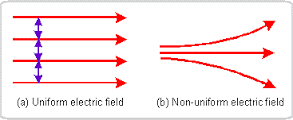Image 2: Uniform and Non-uniform electric field

Torque always acts in a couple, and its magnitude equals to the product of force and its arm. Arm is the distance between the point where the force acts and the point which rotates the dipole. In the dipole placed in the uniform external electric field, we take origin as the point. Torque is denoted by the symbol τ and as it has a direction, it is a vector quantity.

Mathematically,

Magnitude of torque = q E × 2a sin θ

τ = 2 q a E sin θ

τ = p E sin θ (Since p = 2 q a)

The vector form of torque is the cross product of dipole moment and electric field.

To understand what cross product is, let’s take an example.

Example:

Suppose there are two vectors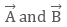then cross product of two vectors will be

Cross product =The direction of cross product is normal to both the vectorsand its magnitude can be evaluated as

Cross product = A B sin θ

where θ is the angle between two vectors.

So, the torque equation in vector form can be written as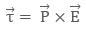Image 3: Torque rotates the dipole in uniform electric field

Observations in net force and torque

Taking the nature of electric field and position of the dipole, following remarks will come out:

If the dipole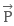and external electric field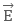are parallel, that is, angle between them is zero, then the dipole will feel zero torque

If the external electric fieldis non-uniform, then net force on the dipole will not be zero, and torque will still act on it

If the dipole external  electric fieldare antiparallel,that is, angle between them is non-zero, then the dipole will feel zero torque

When the electric dipoleand electric fieldare parallel,the direction of net force will be in direction of increasing electric fieldImage 4: Direction of net force depends on orientation of electric dipole

When the electric dipoleand electric fieldare anti-parallel, then the direction of net force will be in direction of decreasing electric field

Force and Torque on a dipole placed in a uniform external fieldvaries with the orientation of dipole in free space

Physical Significance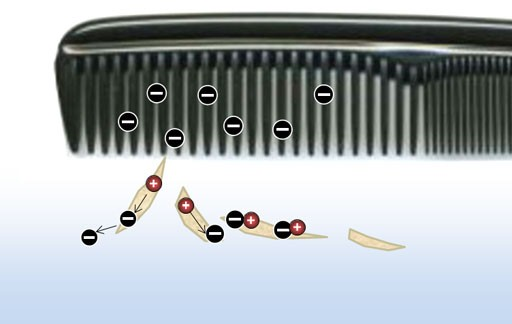When we comb our dry hair and bring it near to some paper pieces, we find that the comb attracts the paper pieces. The comb gains charge, from our hair by the process of rubbing and induce a charge in the uncharged paper. In another way, the comb polarizes the pieces of paper that is, generate a net dipole moment in the direction of electric field. Also since the electric field is non-uniform, the paper pieces move in the direction of the comb.

Watch this Video for more reference

Dipole in Uniform External Field
```### Course Features

• 101 Video Lectures
• Revision Notes
• Previous Year Papers
• Mind Map
• Study Planner
• NCERT Solutions
• Discussion Forum
• Test paper with Video Solution